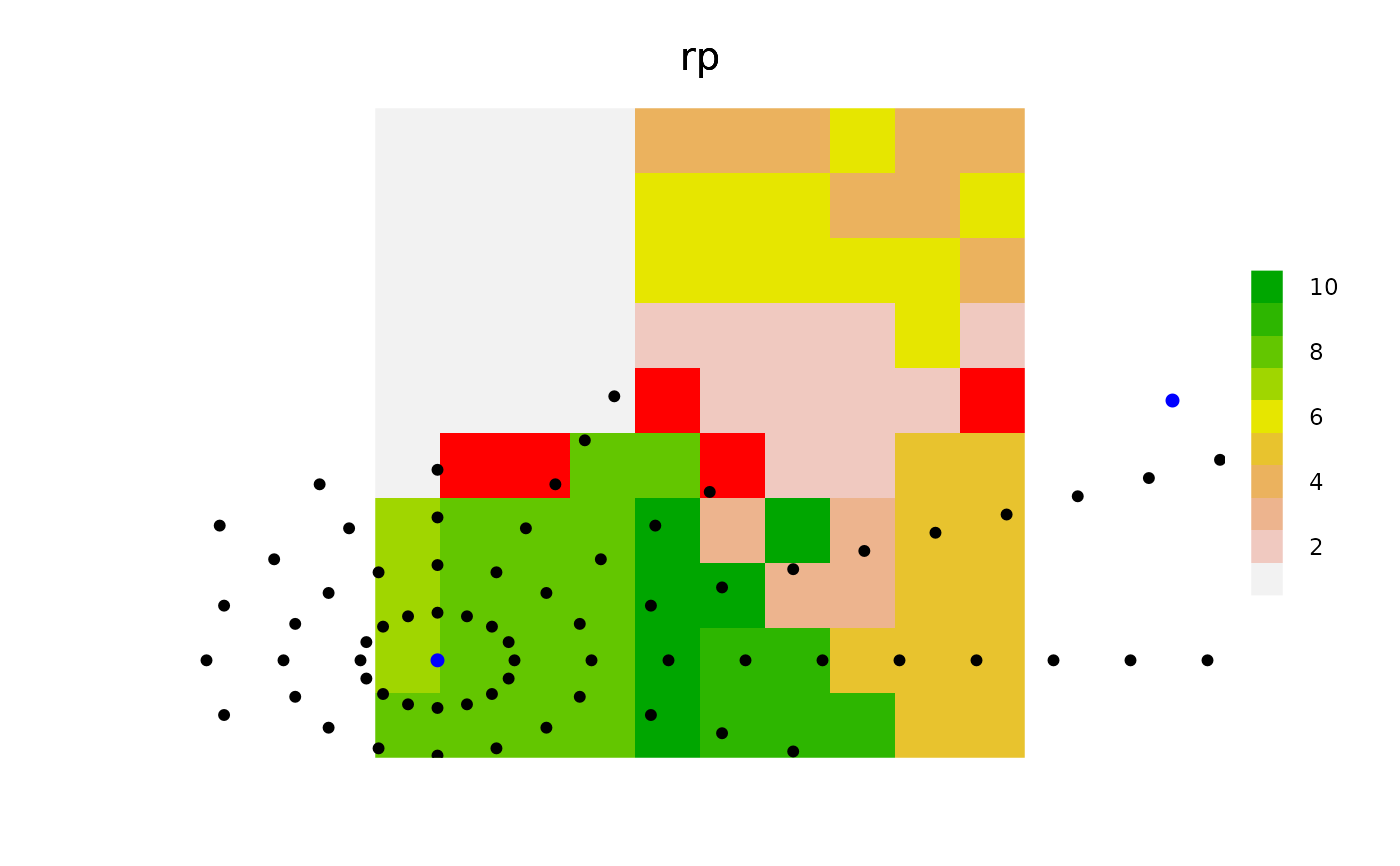This is a generalized version of a notion of a viewshed. The main difference is that there can be many "viewpoints".

spokes(
landscape,
coords,
loci,
allowOverlap = TRUE,
stopRule = NULL,
includeBehavior = "includePixels",
returnDistances = FALSE,
angles = NA_real_,
nAngles = NA_real_,
returnAngles = FALSE,
returnIndices = TRUE,
...
)

# S4 method for RasterLayer,SpatialPoints,missing
spokes(
landscape,
coords,
loci,
allowOverlap = TRUE,
stopRule = NULL,
includeBehavior = "includePixels",
returnDistances = FALSE,
angles = NA_real_,
nAngles = NA_real_,
returnAngles = FALSE,
returnIndices = TRUE,
...
)

## Arguments

landscape

Raster on which the circles are built.

coords

Either a matrix with 2 (or 3) columns, x and y (and id), representing the coordinates (and an associated id, like cell index), or a SpatialPoints* object around which to make circles. Must be same coordinate system as the landscape argument. Default is missing, meaning it uses the default to loci

loci

Numeric. An alternative to coords. These are the indices on landscape to initiate this function. See coords. Default is one point in centre of landscape..

Numeric vector of length 1 or same length as coords

Numeric vector of length 1 or same length as coords. Default is maxRadius, meaning return all cells that are touched by the narrow ring at that exact radius. If smaller than maxRadius, then this will create a buffer or donut or ring.

allowOverlap

Logical. Should duplicates across id be removed or kept. Default TRUE.

stopRule

A function. If the spokes are to stop. This can be a function of landscape, fromCell, toCell, x (distance from coords cell), or any other named argument passed into the ... of this function. See examples.

includeBehavior

Character string. Currently accepts only "includePixels", the default, and "excludePixels". See details.

returnDistances

Logical. If TRUE, then a column will be added to the returned data.table that reports the distance from coords to every point that was in the circle/donut surrounding coords. Default FALSE, which is faster.

angles

Numeric. Optional vector of angles, in radians, to use. This will create "spokes" outward from coords. Default is NA, meaning, use internally derived angles that will "fill" the circle.

nAngles

Numeric, length one. Alternative to angles. If provided, the function will create a sequence of angles from 0 to 2*pi, with a length nAngles, and not including 2*pi. Will not be used if angles is provided, and will show warning of both are given.

returnAngles

Logical. If TRUE, then a column will be added to the returned data.table that reports the angle from coords to every point that was in the circle/donut surrounding coords. Default FALSE.

returnIndices

Logical or numeric. If 1 or TRUE, will return a data.table with indices and values of successful spread events. If 2, it will simply return a vector of pixel indices of all cells that were touched. This will be the fastest option. If FALSE, then it will return a raster with values. See Details.

...

Objects to be used by stopRule(). See examples.

## Value

A matrix containing columns id (representing the row numbers of coords), angles (from coords to each point along the spokes), x and y coordinates of each point along the spokes, the corresponding indices on the landscape

Raster, dists (the distances between each coords and each point along the spokes), and stop, indicating if it was a point that caused a spoke to stop going outwards due to stopRule.

Eliot McIntire

## Examples

library(sp)
library(raster)
library(quickPlot)

set.seed(1234)

ras <- raster(extent(0, 10, 0, 10), res = 1, val = 0)
rp <- randomPolygons(ras, numTypes = 10)

clearPlot()
Plot(rp)

angles <- seq(0, pi * 2, length.out = 17)
angles <- angles[-length(angles)]
n <- 2
loci <- sample(ncell(rp), n)
coords <- SpatialPoints(xyFromCell(rp, loci))
stopRule <- function(landscape) landscape < 3
d2 <- spokes(rp, coords = coords, stopRule = stopRule,
returnAngles = TRUE, returnDistances = TRUE,
allowOverlap = TRUE, angles = angles, returnIndices = TRUE)

# Assign values to the "patches" that were in the viewshed of a ray
rasB <- raster(ras)
rasB[] <- 0
rasB[d2[d2[, "stop"] == 1, "indices"]] <- 1

Plot(rasB, addTo = "rp", zero.color = "transparent", cols = "red")

if (NROW(d2) > 0) {
sp1 <- SpatialPoints(d2[, c("x", "y")])
Plot(sp1, addTo = "rp", pch = 19, size = 5, speedup = 0.1)
}
Plot(coords, addTo = "rp", pch = 19, size = 6, cols = "blue", speedup = 0.1)clearPlot()